# 1.本周学习总结

## ASL

### 顺序查找

ASL（成功）：(1+2+ ... +n)/n=(n+1)/2
ASL（不成功）：n

### 二分查找(折半查找）

• 将表中间位置记录的关键字与查找关键字比较，如果两者相等，则查找成功；
• 否则利用中间位置记录将表分成前、后两个子表，如果中间位置记录的关键字大于查找关键字，则进一步查找前一子表，否则进一步查找后一子表。
• 重复以上过程，直到找到满足条件的记录，使查找成功，或直到子表不存在为止，此时查找不成功。
• 二分查找要求线性表必须采用顺序存储结构，而且表中元素按关键字有序排列
ASL(成功）=$$log_2{(n+1)} -1$$
ASL(不成功）=

## 二叉搜索(BST)

• 若它的左子树不空，则左子树上所有结点的值均小于它的根结点的值
• 若它的右子树不空，则右子树上所有结点的值均大于它的根结点的值
• 它的左、右子树也分别为二叉搜索树
• 按中序遍历BST所得到的中序序列是一个递增有序序列

typedef struct BSTNode
{
KeyType key; //数据域
BSTNode *lchild;
BSTNode *rchild;
}

### 构建与插入

BSTNode *CreateBST(KeyType A[], int n)
{
BSTNode *bt=NULL;
int i=0;
while(i<n)
{
InsertBST(bt, A[i]);
i++;
}
return bt;
}

int InsertBST(BSTNode *p, KeyType k)
{
if(p==NULL)
{
p=(BSTNode*)malloc(sizeof(BSTNode));
p->key=k;
p->lchild=p->rchild=NULL;
return 1;
}
else if(k==p->key)
return 0;
else if(k<p->key)
return InsertBST(p->lchild, k);
else
return InsertBST(p->rchild, k);
}


### 查找

#### 递归形式

BSTree SearchBST(BSTree t, int k)
{
if(t==null || k==t->key)
return t;
else if(k<t->key)
return SearchBST(t->lchild, k);
else
return SearchBST(t->rchild, k);
}


### 非递归形式

BSTree SearchBST2(BSTree t, int k)
{
BSTree p=t;
while(p!=null && p->key!=k)
{
if(k<p->key)
p=p->lchild;
else
p=p->rchild;
}
return p;
}


### 删除

void DelBSTNode( BiTree t, int key )
{
BiTree p, q;
p = t;
int temp;
while( NULL != p && key != p->data )
{
q = p;
if( key < p->data )
p = p->lchild ;
else
p = p->rchild ;
}

if( NULL == p )
printf("无此元素!\n");
else {
//情况1:结点p的双亲结点为q，且p为叶子结点，则直接将其删除。
if( NULL == p->lchild && NULL == p->rchild )
{
if( p == q->lchild )
q->lchild = NULL;
if( p == q->rchild )
q->rchild = NULL;
free(p);
p = NULL;
}
//情况2:结点p的双亲结点为q，且p只有左子树或只有右子树，则可将p的左子树或右子树直接改为其双亲结点q的左子树或右子树。
else if( (NULL == p->rchild && NULL != p->lchild) ) //p只有左子树
{
if( p == q->lchild )
q->lchild = p->lchild ;
else if( p == q->rchild )
q->rchild = p->lchild ;
free(p);
p = NULL;
}
else if( NULL == p->lchild && NULL != p->rchild ) //p只有右子树
{
if( p == q->lchild )
q->lchild = p->rchild ;
if( p == q->rchild )
q->rchild = p->rchild ;
free(p);
p = NULL;
}
//情况3:结点p的双亲结点为q，且p既有左子树又有右子树。
else if( NULL != p->lchild && NULL != p->rchild )
{
BiTree s, sParent;
sParent = p;
s = sParent->lchild ;
while( NULL != s->rchild )
{
//找到p的直接前驱
sParent = s;
s = s->rchild ;
}
temp = s->data ;
DelBSTNode( t, temp );
p->data = temp;
}
}
}


## AVL树

• 本身首先是一棵二叉搜索树
• AVL树中任何节点的两个子树的高度最大差别为1，所以它也被称为高度平衡树

### LL型调整

1、 将根节点的左孩子作为新根节点。
2、 将新根节点的右孩子作为原根节点的左孩子。
3、 将原根节点作为新根节点的右孩子。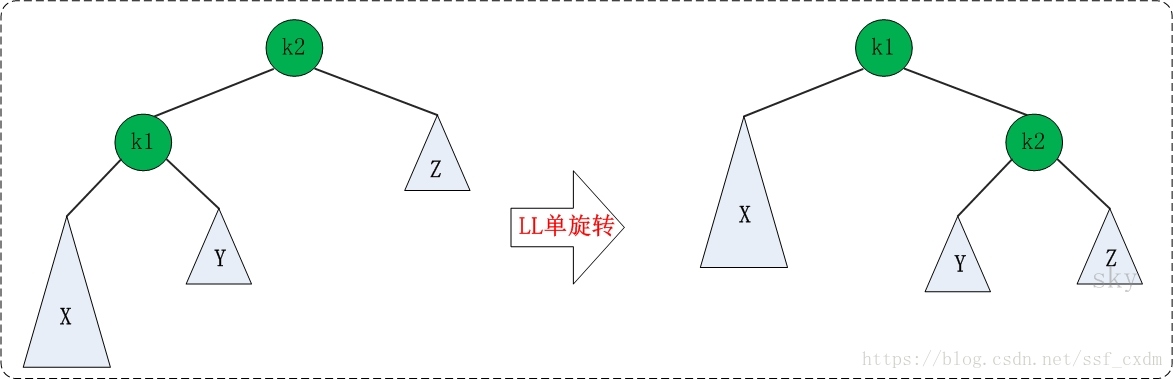### RR型调整

1、 将根节点的右孩子作为新根节点。
2、 将新根节点的左孩子作为原根节点的右孩子。
3、 将原根节点作为新根节点的左孩子。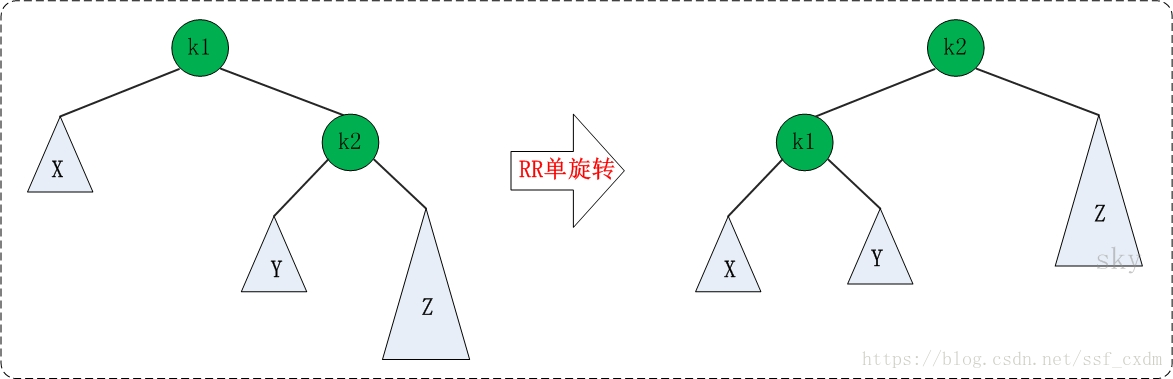### LR型调整

1、 围绕根节点的左孩子进行RR旋转。
2、 围绕根节点进行LL旋转。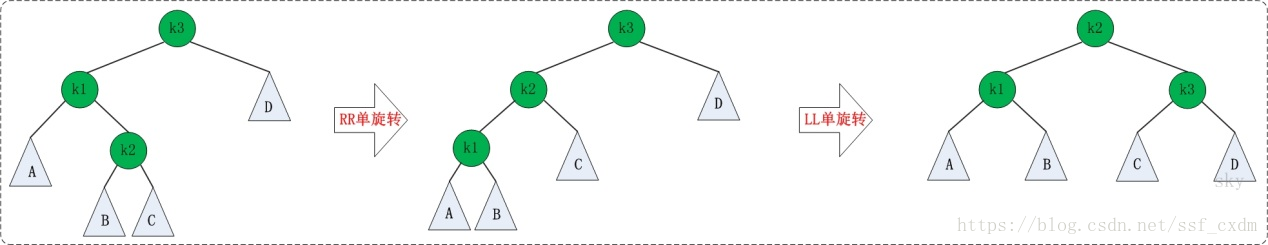### RL型调整

1、 围绕根节点的右孩子进行LL旋转。
2、 围绕根节点进行RR旋转。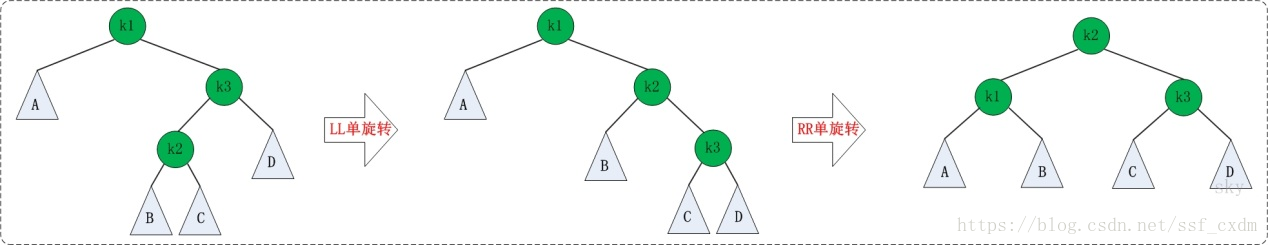## B-树

B-树是一种多路搜索树（并不一定是二叉的）
1、 根结点至少有两个子女；
2、 每个非根节点所包含的关键字个数 j 满足：┌m/2┐ - 1 <= j <= m - 1；
3、 除根结点以外的所有结点（不包括叶子结点）的度数正好是关键字总数加1，故内部子树个数 k 满足：┌m/2┐ <= k <= m ；
4、 所有的叶子结点都位于同一层。

### 插入

1、 如果该结点的关键字个数未到达m-1个，那么直接插入；
2、 如果该结点的关键字个数已到达m-1个，那么根据B树的性质显然无法满足，需要将其进行分裂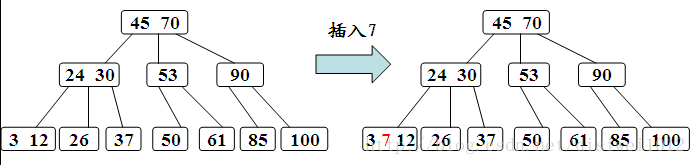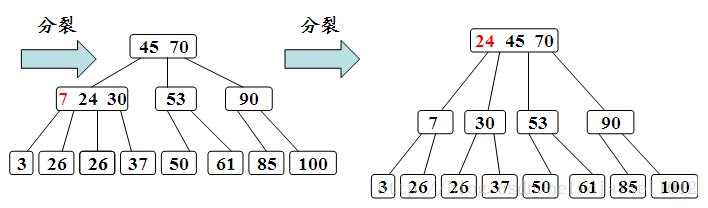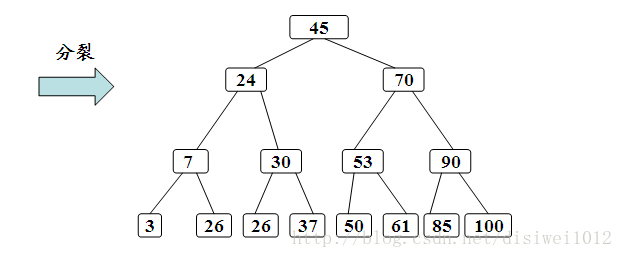### 删除

1、 被删关键字所在结点中的关键字个数>=[m/2],说明删去该关键字后该结点仍满足B-树的定义，只需从该结点中直接删去关键字即可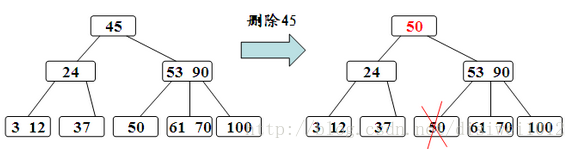2、 被删关键字所在结点中的关键字个数=[m/2]-1，说明删去该关键字后该结点将不满足B-树的定义，需要调整。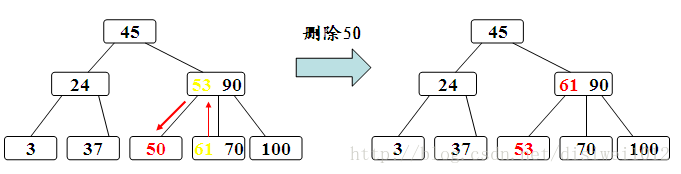3、 被删关键字所在结点其相邻的左右兄弟节点中的关键字个数均等于[m/2]-1，左右兄弟都不够借。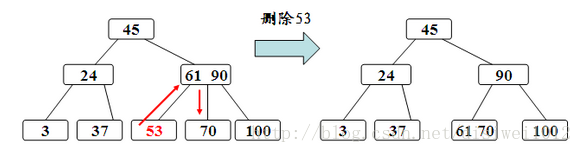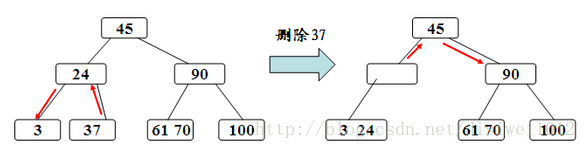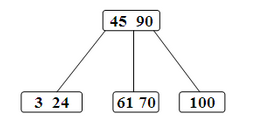## B+树

B+树的特点是能够保持数据稳定有序，其插入与修改拥有较稳定的对数时间复杂度。B+树元素自底向上插入。
1、 根结点至少有两个子女。
2、 每个中间节点都至少包含ceil(m / 2)个孩子，最多有m个孩子。
3、 每一个叶子节点都包含k-1个元素，其中 m/2 <= k <= m。
4、 所有的叶子结点都位于同一层。
5、 每个节点中的元素从小到大排列，节点当中k-1个元素正好是k个孩子包含的元素的值域分划。

## 哈希表

• 哈希函数h(key)：把关键字为ki的对象存放在相应的哈希地址中
• 哈希表：存储数据记录的长度为m（m≥n）的连续内存单元
• 装填因子α=存储的记录个数/哈希表的大小=n/m，α越小，冲突可能性就越小； α越大（最大可取1），冲突的可能性就越大。控制在0.6～0.9的范围内

### 哈希函数构造

#### 直接定址法

• h(k)=k+c
• 优点：计算简单,并且不可能有冲突发生
• 缺点：关键字分布不连续将造成内存单元的大量浪费

#### 除留余数法

• h(k)=k mod p (mod为求余运算，p≤m),p与m不一定相同，p最好是质数（素数）

### 哈希冲突

#### 线性探查法

d0=h(k)
di=(di-1+1) mod m (1≤i≤m-1)

#### 平方探查法

d0=h(k)
di=(d0± i2) mod m (1≤i≤m-1)

### ASL

ASL(成功)=每个元素探测次数之和/哈希表元素个数
ASL(不成功)=探查失败次数之和/哈希表元素个数

# 2.对查找的认识及学习体会

## 2.1 7-1 是否完全二叉搜索树

### 2.1.2 伪代码

#include<iostream>
#include<queue>
using namespace std;
typedef struct TNode* Position;
typedef Position BinTree;
struct TNode {
int Data;
BinTree Left;
BinTree Right;
};
BinTree Insert(BinTree& T, int k);
bool Level(BinTree T);
int main()
{
BinTree T;
int N,k;
T = NULL;
cin >> N;
while (N)
{
建树;
}
if (层次遍历并判断) cout<<"YES";
else cout<<"NO";
return 0;
}


### 2.1.3 PTA提交列表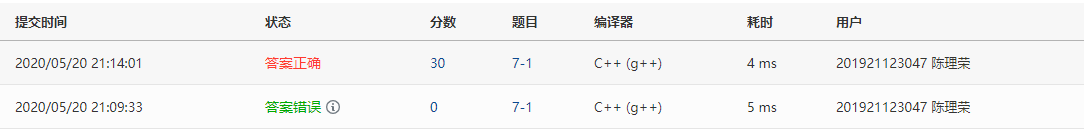## 2.2 7-4 整型关键字的散列映射

### 2.2.2 伪代码

#include <iostream>
using namespace std;
#define MaxSize 1002
#define NULLKEY -1
#define DELKEY -2
typedef char* InfoType;
typedef struct {
int key;// 关键字域
InfoType data;//其他数据域
int count; //探查次数
}HashTable[MaxSize];

void InsertHT(HashTable ha, int& n, int k, int p);
void CreateHT(HashTable ha, int x[], int n, int m, int p);
int SearchHT(HashTable ha, int p, int k);
int main()
{
int x[MaxSize];
int i, N, P, m = 0;
HashTable ha;
cin >> N >> P;
for (i = 0; i < N; i++)
cin >> x[i];
CreateHT(ha, x, N, m, P);
return 0;
}
void InsertHT(HashTable ha, int& n, int k, int p)
{
if (出现重复关键字)return;
if (地址为空，可插入数据)
{
插入并输出;
}
else
{
i = 1;
while (地址不空)
{
线性探查法;
}
插入并输出;
}
n++;
}
void CreateHT(HashTable ha, int x[], int n, int m, int p)
{
int i, j;
初始化哈希表;
for (i = 0; i < n; i++)
{
InsertHT(ha, m, x[i], p);
}
}


### 2.2.3 PTA提交列表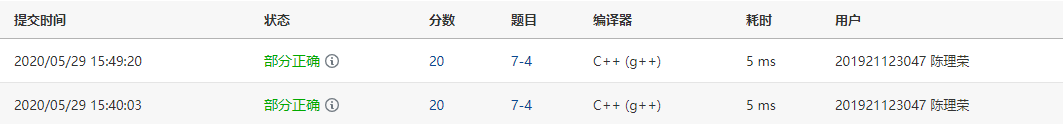• 除留余数法构造哈希函数
• 线性探查法解决哈希冲突

## 2.3 7-5(哈希链) 航空公司VIP客户查询

### 2.3.2 伪代码

#include <iostream>
#include<string>
using namespace std;
#define MAX 1001
typedef struct HashNode
{
string  name;
int kilo;
struct HashNode* next;
}HashNode, * HashTable;
typedef struct hashList
{
int length;
HashTable* ha;
}*Hash;
Hash CreateHash(int N);
void InsertHash(Hash h, string NAME, int K);
int FindHash(Hash h, string NAME, int K);
int main()
{
int N, K, i, num,M;
string NAME,custom;
Hash h;
cin >> N >> K;
h=CreateHash(N);
for (i = 0; i < N; i++)
{
建哈希链
}
cin >> M;
for (i = 0; i < M; i++)
{
cin >> custom;
计算里程，非会员返回-1;
输出;
}
return 0;
}


### 2.3.3 PTA提交列表### 2.3.4 本题设计的知识点

• 哈希函数的设计
• 如何降低时间复杂度
posted @ 2020-05-24 21:07  极仙  阅读(188)  评论(0编辑  收藏  举报